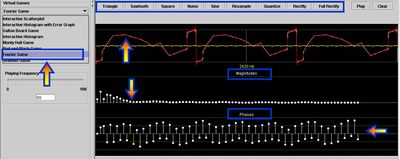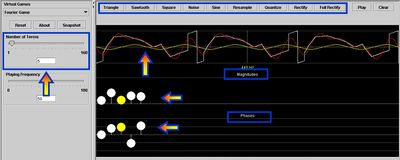# SOCR EduMaterials GamesActivitiesFourier

## SOCR_EduMaterials_GamesActivities Fourier Game

### Summary

This activity represents a general demonstration of the effects of the Fourier Transform on different 1D periodic signals.

### Goals

The aims of this activity are to

• demonstrate the spectral (frequency-space) representation of data (signals) using the Fourier base functions (sines and cosines)
• illustrate the effects of manually manipulating the magnitudes and the phases of the Fourier coefficients on the original signal
• justify the process of Fourier-space thresholding (filtering) as a tool for:
• signal denoising
• increasing the power of statistical analyses in the wavelet domain
• demonstrate the infinite support of the Fourier base functions and their global effects.

### The SOCR Fourier Game

To start this Game, go to SOCR Games and select the Fourier Game from the drop-down list of games in the left panel. The image below shows the interface to this game. You can select from a set of predefined periodic functions or click on the top graph panel, drag and draw a new function (signal) using the mouse. Notice the Fourier representation of the signal on the bottom two panels in terms of the magnitudes and phases.### Experiment 1

Now we will investigate the properties of Fourier filtering and the effects of the position, magnitude and phases of the Fourier coefficients. Bring the mouse over any of the Fourier coefficients in either of the 2 bottom graph panels. You will see a yellow-color plot in the top panel superimposed on top of the original signal. This demonstrates the frequency and phase of the corresponding Fourier base function, whose coefficient you have selected (by the mouse over). Now change the number of Fourier coefficients that are used to reconstruct (synthesize) the signal (choose 5 terms, using the slider on the left). This will show you a red-color curve that is in the same space as the original signal and represents a wavelet-based signal approximation. Lastly, click on any of the large Fourier coefficients (middle graph panel) or any of the phases (bottom panel) and change its magnitude. Notice the effect of this manipulation on the approximation on the original signal (location, frequency and extend of this effect) on the top panel.

Try to pose and answer questions like: If you set to zero the Fourier coefficients in the right (or left) end of the Fourier domain, what will be the effect on the signal in time/space?